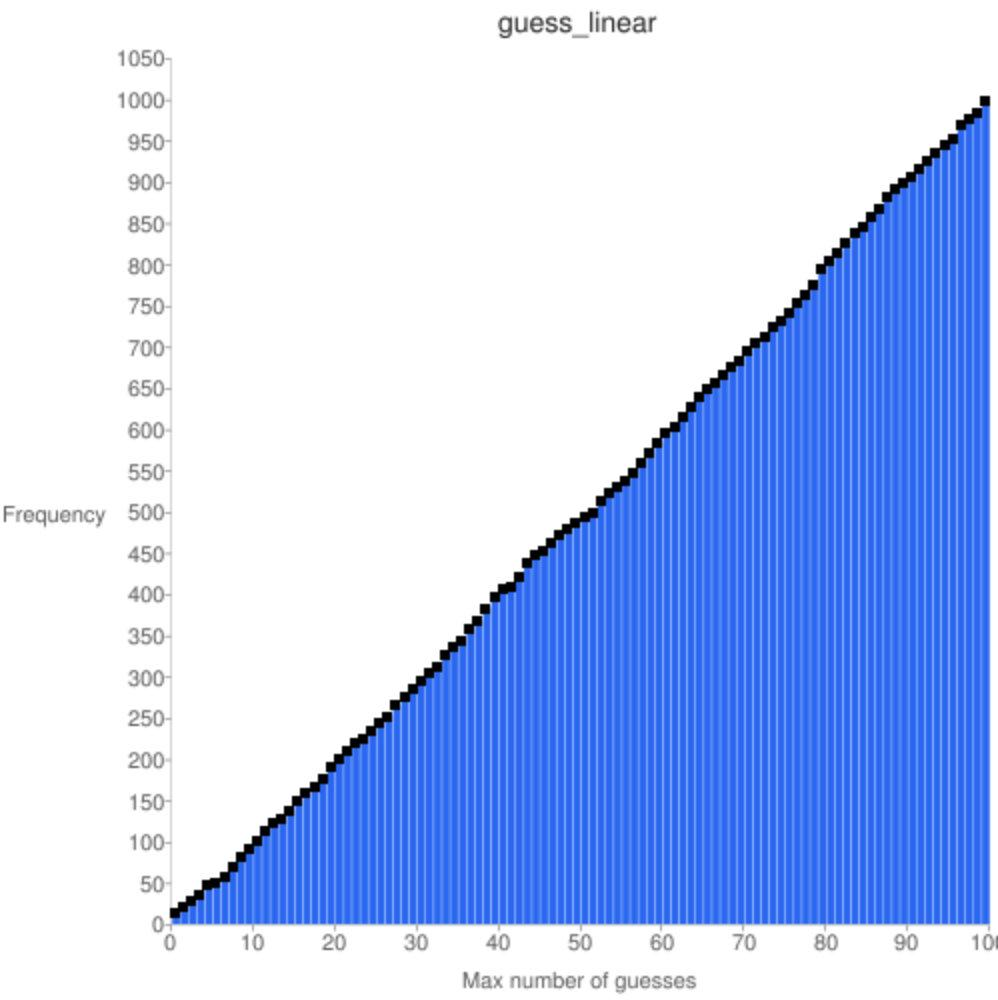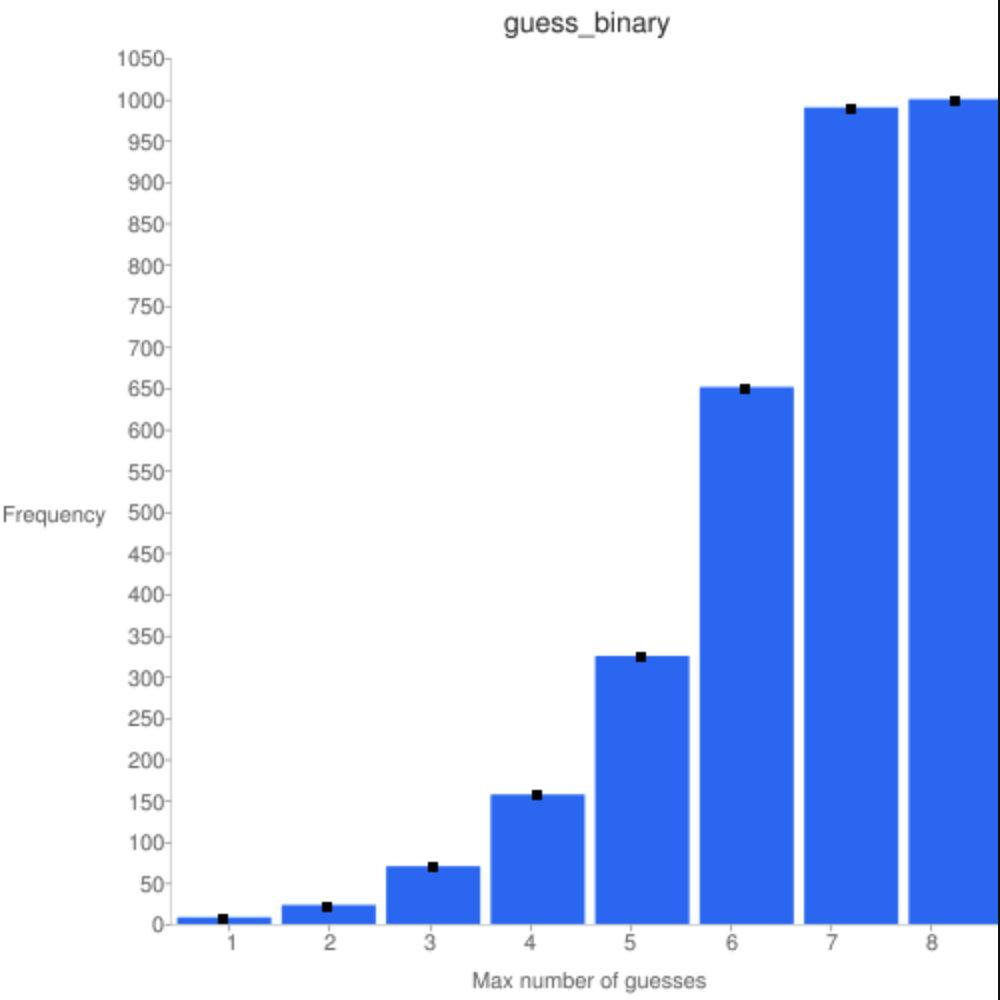# 日拱一卒，一起來上伯克利的實驗課，帶你入門Python

Github

https://inst.eecs.berkeley.edu//~cs61a/sp18/lab/lab01/#using-python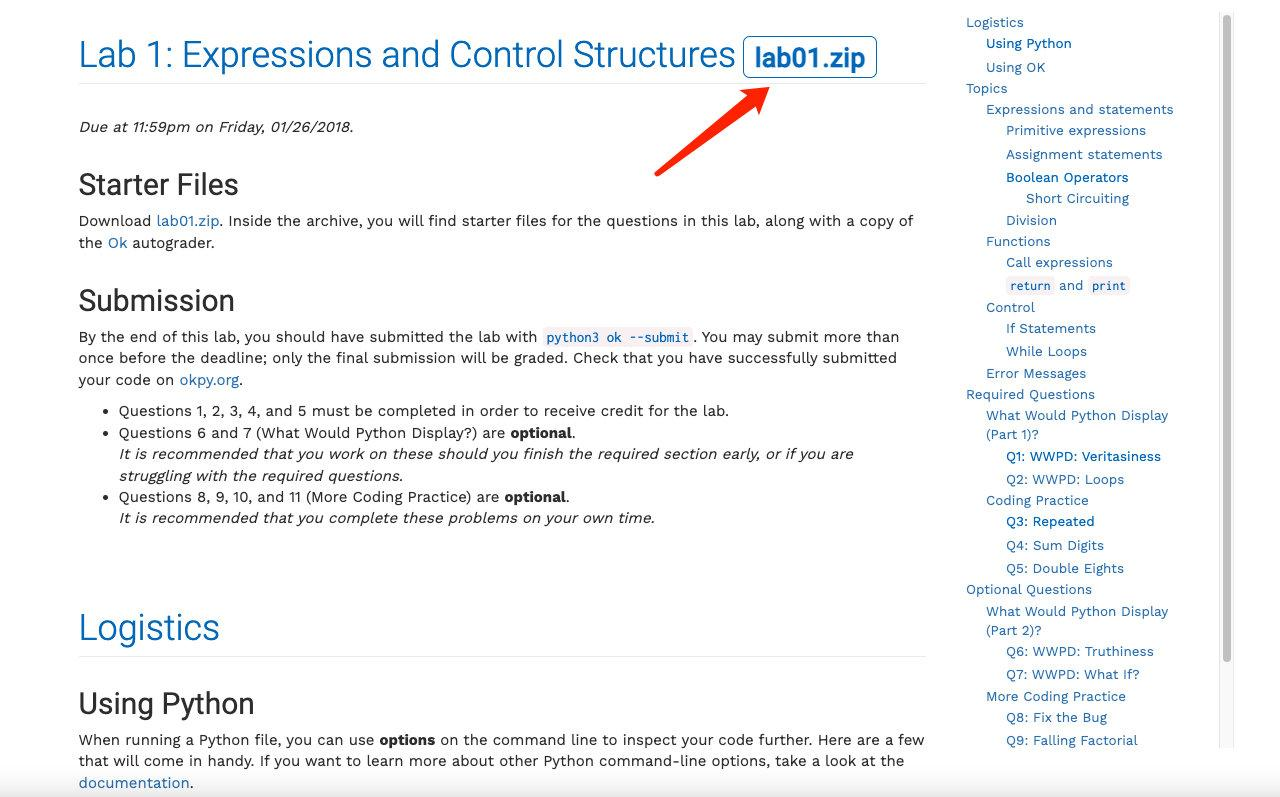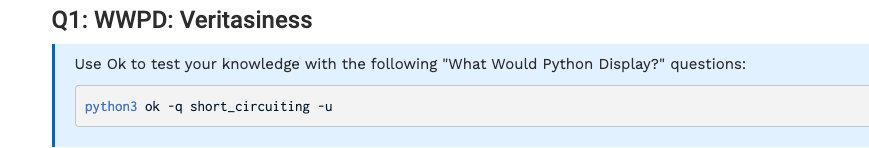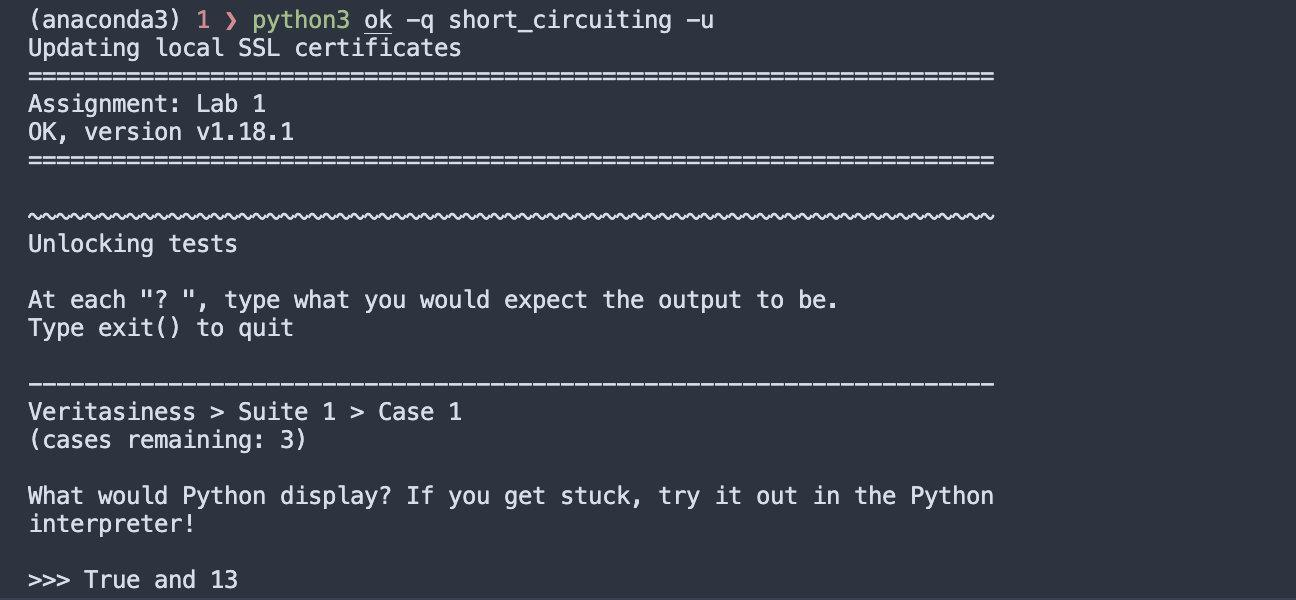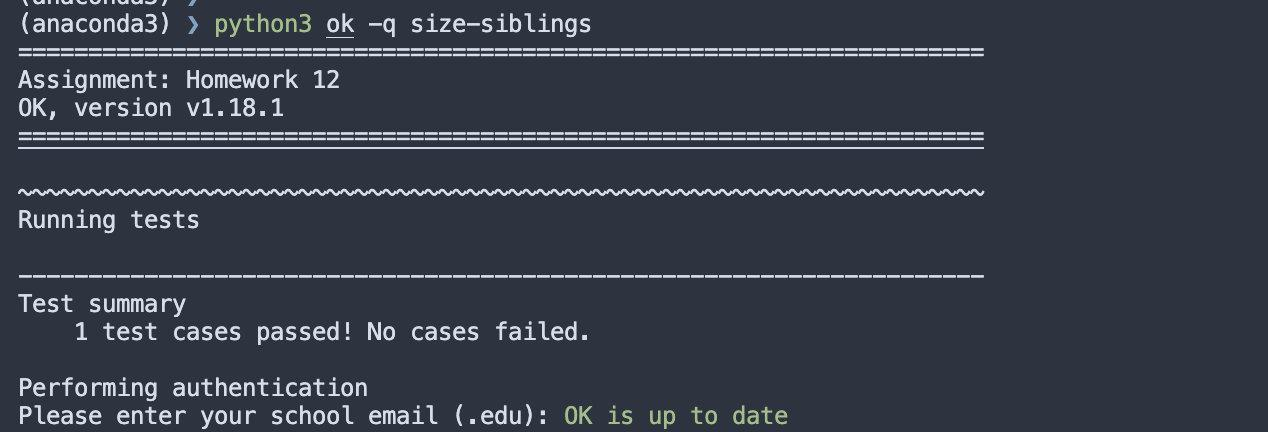## What Would Python Display (Part 1)?

### Q1: WWPD: Veritasiness

```python

True and 13

False or 0

not 10

not None

True and 1 / 0 and False

True or 1 / 0 or False

True and 0

False or 1

1 and 3 and 6 and 10 and 15

0 or False or 2 or 1 / 0

not 0

(1 + 1) and 1

1/0 or True

(True or False) and False

```

### Q2: WWPD: Loops

```python

n = 3 while n >= 0: ... n -= 1 ... print(n)

n = 4 while n > 0: ... n += 1 ... print(n)

def go(n): ... if n % 2 != 0: ... print(n / 2) ... return ... while n > 0: ... print(n) ... n = n // 2 go(4)

go(5)

zero = 2 while zero != 0: ... zero = zero // 2 ... print(zero)

positive = 28 while positive: ... print("positive?") ... positive -= 3

positive = -9 negative = -12 while negative: ... if positive: ... print(negative) ... positive += 3 ... negative += 3

```

## Coding Practice

### Q3: Repeated

```python

def square(x): ... return x * x ... repeated(square, 2, 3) # square(square(3)), or 3 ** 4 81 repeated(square, 1, 4) # square(4) 16 repeated(square, 6, 2) # big number 18446744073709551616 def opposite(b): ... return not b ... repeated(opposite, 4, True) True repeated(opposite, 5, True) False repeated(opposite, 631, 1) False repeated(opposite, 3, 0) True """ ```

#### 答案

```python def repeated(f, n, x): """Returns the result of composing f n times on x. """ "*** YOUR CODE HERE ***" if n == 1: return f(x) return f(repeated(f, n-1, x))```

```python def repeated(f, n, x): """Returns the result of composing f n times on x. """ "*** YOUR CODE HERE ***" ret = x for _ in range(n): ret = f(ret) return ret```

### Q4: Sum Digits

```python

sum_digits(10) # 1 + 0 = 1 1 sum_digits(4224) # 4 + 2 + 2 + 4 = 12 12 sum_digits(1234567890) 45 ```

#### 答案

```python def sum_digits(n): """Sum all the digits of n. """ "*** YOUR CODE HERE ***" if n < 10: return n return sum_digits(n // 10) + n % 10```

```python def sum_digits(n): """Sum all the digits of n. """ "*** YOUR CODE HERE ***" ret = 0 while n > 0: ret += n % 10 n //= 10 return ret```

### Q5: Double Eights

```python

double_eights(8) False double_eights(88) True double_eights(2882) True double_eights(880088) True double_eights(12345) False double_eights(80808080) False ```

#### 答案

```python def double_eights(n): """Return true if n has two eights in a row. """ "*** YOUR CODE HERE ***" return '88' in str(n)```

```python def double_eights(n): """Return true if n has two eights in a row. """ "*** YOUR CODE HERE ***" while n > 10: if n % 100 == 88: return True n //= 10 return False```

```python def double_eights(n): """Return true if n has two eights in a row. """ "*** YOUR CODE HERE ***" if n < 10: return False pref, suff = (n % 100) // 10, n % 10 return (pref == 8 and suff == 8) or double_eights(n // 10)```

# 附加題

## What Would Python Display (Part 2)?

### Q6: WWPD: Truthiness

```python

0 or True

not '' or not 0 and False

13 and False

False or 1

'' or 1 and 1/0

not 0 and 12 or 0

```

### Q7: WWPD: What If?

```python

def xk(c, d): ... if c == 4: ... return 6 ... elif d >= 4: ... return 6 + 7 + c ... else: ... return 25 xk(10, 10)

xk(10, 6)

xk(4, 6)

xk(0, 0)

def how_big(x): ... if x > 10: ... print('huge') ... elif x > 5: ... return 'big' ... elif x > 0: ... print('small') ... else: ... print("nothin'") how_big(7)

how_big(12)

how_big(1)

how_big(-1)

def so_big(x): ... if x > 10: ... print('huge') ... if x > 5: ... return 'big' ... if x > 0: ... print('small') ... print("nothin'") so_big(7)

so_big(12)

so_big(1)

def ab(c, d): ... if c > 5: ... print(c) ... elif c > 7: ... print(d) ... print('foo') ab(10, 20)

def bake(cake, make): ... if cake == 0: ... cake = cake + 1 ... print(cake) ... if cake == 1: ... print(make) ... else: ... return cake ... return make bake(0, 29)

bake(1, "mashed potatoes")

```

## More Coding Practice

### Q8: Fix the Bug

```python def both_positive(x, y): """Returns True if both x and y are positive.

``````>>> both_positive(-1, 1)
False
>>> both_positive(1, 1)
True
"""
return x and y > 0 # You can replace this line!
``````

```

### Q9: Falling Factorial

```python

falling(6, 3) # 6 * 5 * 4 120 falling(4, 0) 1 falling(4, 3) # 4 * 3 * 2 24 falling(4, 1) # 4 4 ```

#### 答案

```python def falling(n, k): """Compute the falling factorial of n to depth k. """ "*** YOUR CODE HERE ***" ret = 1 for i in range(n, n-k, -1): ret *= i return ret```

```python def falling(n, k): """Compute the falling factorial of n to depth k. """ "*** YOUR CODE HERE ***" if k == 0: return 1 return n * falling(n-1, k-1)```

## I Want to Play a Game

`guess_random`函式會讓你先選一個數，然後迴圈你若干次它的猜測是否正確。如果它猜對了，輸入`y`，否則輸入`n`。Python並不擅長猜數，所以可能會猜很久，你可以通過`Ctrl-C`來終止程式。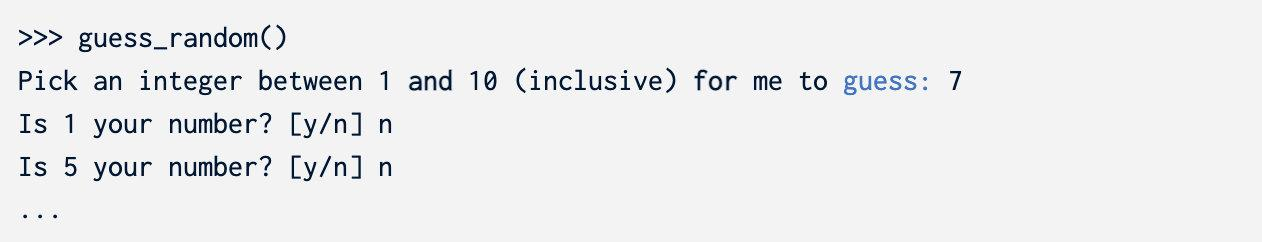### Q10: Guess Linear

`guess_random`策略的一個弱點就是它可能會重複猜測某些錯誤的數字，所以我們可以使用線性猜測讓它更加合理。

```python def guess_linear(): """Guess in increasing order and return the number of guesses.""" prompt_for_number(LOWER, UPPER) num_guesses = 1 guess = LOWER "*** YOUR CODE HERE ***" return num_guesses```

#### 答案

```python def guess_linear(): """Guess in increasing order and return the number of guesses.""" prompt_for_number(LOWER, UPPER) num_guesses = 1 guess = LOWER "*** YOUR CODE HERE ***" while guess <= UPPER: correct = is_correct(guess) if correct: break guess += 1 num_guesses += 1 return num_guesses```

### Q11: Guess Binary

`is_too_high`的用法如下：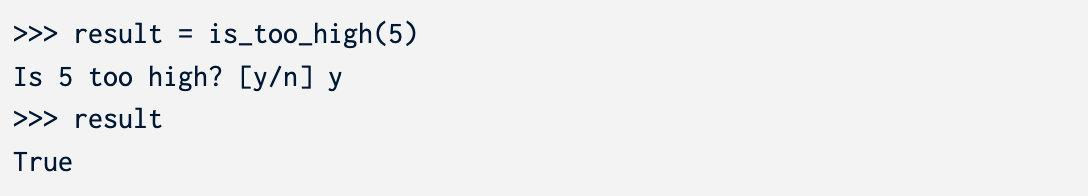```python def guess_binary(): """Return the number of attempted guesses. Implement a faster search algorithm that asks the user whether a guess is less than or greater than the correct number.

``````Hint: If you know the guess is greater than the correct number, then your
algorithm doesn't need to try numbers that are greater than guess.
"""
prompt_for_number(LOWER, UPPER)
num_guesses = 1
lower, upper = LOWER, UPPER
guess = (lower + upper) // 2
return num_guesses
``````

```

### A Second Look

```bash python3 guessing_game_graph.py guess_linear python3 guessing_game_graph.py guess_binary```